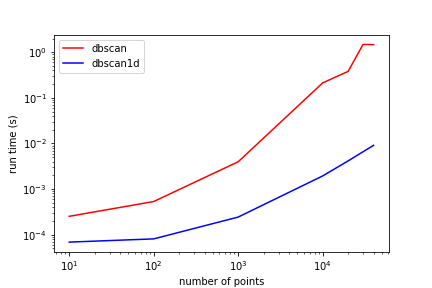An efficient implementation of the DBSCAN algorithm for 1D arrays.

# DBSCAN1D

dbscan1d is a 1D implementation of the DBSCAN algorithm. It was created to efficiently preform clustering on large 1D arrays.

Sci-kit Learn's DBSCAN implementation does not have a special case for 1D, where calculating the full distance matrix is wasteful. It is much better to simply sort the input array and performing efficient bisects for finding closest points. Here are the results of running the simple profile script included with the package. In every case DBSCAN1D is much faster than scikit learn's implementation.## Installation

Simply use pip to install dbscan1d:

pip install dbscan1d


It only requires numpy.

## Quickstart

dbscan1d is designed to be interchangable with sklearn's implementation in almost all cases. The exception is that the weights parameter is not yet supported.

from sklearn.datasets import make_blobs

from dbscan1d.core import DBSCAN1D

# make blobs to test clustering
X = make_blobs(1_000_000, centers=2, n_features=1)

# init dbscan object
dbs = DBSCAN1D(eps=.5, min_samples=4)

# get labels for each point
labels = dbs.fit_predict(X)

# show core point indices
dbs.core_sample_indices_

# get values of core points
dbs.components_


## Notes

• dbscan1d can return different group numbers than sklearn for non-core points which are within eps distances of core points for two separate groups. For example: --C1--C1--P--C2--C2 Here C1 and C2 are core points for group 1 and group 2, respectively. If P is within eps of both C1 and C2, dbscan1d will assign it the same label as the core point that is closest. Sklearn doesn't always do this.

## Project details

This version0.2.20.2.10.1.60.1.40.1.30.1.20.1.10.1.0

Uploaded source
Uploaded py3